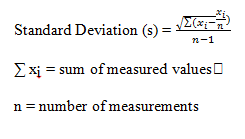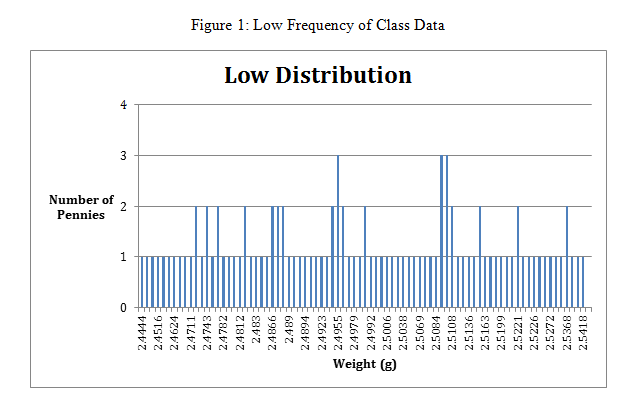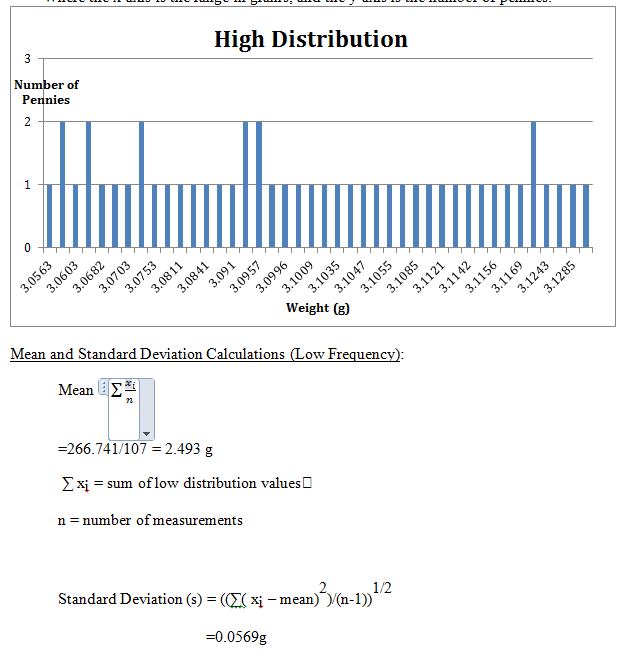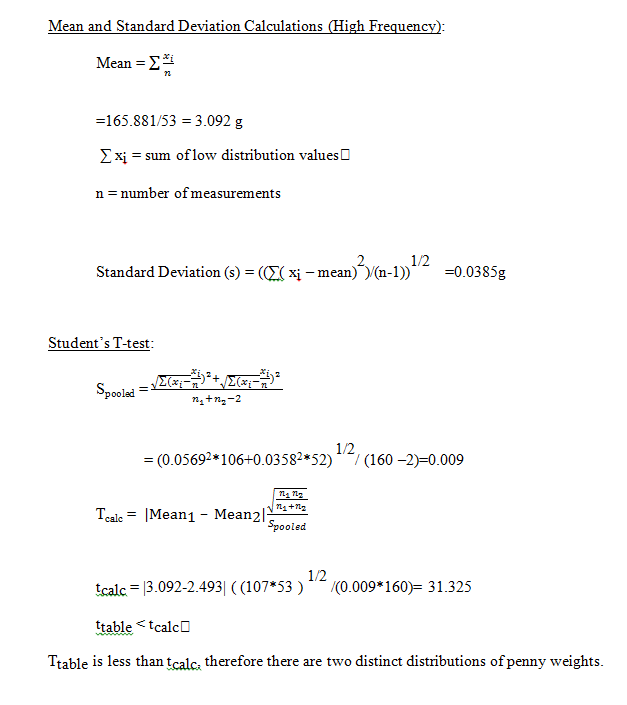# Penny Pinching: Statistical Treatment of Data

By: Julie Wilhelmsen

Objective:

The objective of experiment one is to see if the year a penny is produced in mint, relates to the weight of the penny. Each lab group had a set of 20 pennies, in which we collected the weight and the year of each penny. The information obtained from each lab group will help us create some statistical data. By using different calculations, I will be able to determine if there is a relation between a penny’s weight and year minted. The calculations I will use to do this are: mean, standard deviation, Q-test, and t-test. I predict that pennies that are newly produced will weigh less than pennies produced many years ago.

Data:

Table 1: Individual Lab Group Data

 Penny # Year Mass (g) 1 1980 3.0841 2 2005 2.4948 3 1982 3.0996 4 1996 2.5038 5 1993 2.4829 6 1988 2.4724 7 1984 2.5723 8 1995 2.5191 9 1990 2.5269 10 1979 3.1068 11 1999 2.4890 12 1995 2.4901 13 1984 2.5202 14 1988 2.5154 15 1983 2.5108 16 1974 3.0823 17 1991 2.4502 18 1993 2.4807 19 1985 2.4657 20 1963 3.0910

Table 2: Class Data

 Penny # Year Mass (g) Penny # Year Mass (g) 1 1988 2.4444 41 1994 2.4624 2 1993 2.5002 42 1996 2.4743 3 1990 2.4990 43 1991 2.5226 4 1990 2.4711 44 1979 3.0753 5 1959 3.0603 45 1968 3.0454 6 1994 2.4732 46 1990 2.4991 7 1993 2.4857 47 1995 2.4516 8 2001 2.4843 48 1985 2.5118 9 1993 2.5222 49 2005 2.5108 10 2001 2.5035 50 1969 3.0703 11 1982 3.1035 51 1972 3.0694 12 1974 3.1047 52 1984 2.483 13 1989 2.5413 53 1996 2.4724 14 1989 2.4786 54 1982 3.116 15 1986 2.4923 55 1982 3.1009 16 1982 3.0563 56 1987 2.5218 17 1988 2.4533 57 1999 2.4892 18 1973 3.1142 58 1988 2.4492 19 1964 3.1085 59 1987 2.5223 20 1972 3.1025 60 1994 2.5136 21 1981 3.0091 61 1979 3.1048 22 1991 2.5374 62 1988 2.5082 23 1986 2.5418 63 1999 2.5111 24 1978 3.1921 64 1995 2.4893 25 2006 2.4812 65 1970 3.1001 26 1982 3.1121 66 2005 2.4979 27 1980 3.1286 67 1996 2.5063 28 1975 3.1243 68 1977 3.1096 29 1996 2.5083 69 1989 2.4948 30 2005 2.5084 70 1975 3.1283 31 1995 2.5006 71 1983 2.5272 32 1978 3.1169 72 1973 3.0811 33 1982 3.096 73 1995 2.4991 34 1959 3.1042 74 1978 3.1055 35 1980 3.0782 75 1989 2.5331 36 1989 2.4955 76 1982 3.1122 37 1990 2.5159 77 1986 2.4782 39 1993 2.5036 79 2003 2.5107 40 2001 2.4992 80 1994 2.517 Penny # Year Mass (g) Penny # Year Mass (g) 81 1987 2.4966 121 1994 2.4963 82 1984 2.5722 122 1992 2.5107 83 1990 2.5288 123 1977 3.1174 84 1984 2.5001 124 2003 2.4819 85 1973 3.0682 125 1968 3.0723 86 1991 2.5163 126 2000 2.4884 87 2000 2.4757 127 1996 2.4955 88 1983 2.5606 128 1998 2.4758 89 1990 2.5261 129 1974 3.0957 90 1988 2.4743 130 2001 2.4866 91 1990 2.4639 131 1982 3.115 92 2001 2.5159 132 1979 3.0664 93 1986 2.5069 133 1988 2.9095 94 1989 2.4943 134 1992 2.4875 95 1996 2.4903 135 1975 3.1285 96 1975 3.1156 136 1984 2.5368 97 1986 2.5199 137 1963 3.0583 98 1980 3.1549 138 1994 2.5095 99 1990 2.4611 139 1998 2.5221 100 1997 2.5042 140 1980 3.0947 101 1980 3.0841 141 1994 2.4963 102 2005 2.4948 142 1992 2.5107 103 1982 3.0996 143 1977 3.1174 104 1996 2.5038 144 2003 2.4819 105 1993 2.4829 145 1968 3.0723 106 1988 2.4724 146 2000 2.4884 107 1984 2.5723 147 1996 2.4955 108 1995 2.5191 148 1998 2.4758 109 1990 2.5269 149 1974 3.0957 110 1979 3.1068 150 2001 2.4866 111 1999 2.489 151 1982 2.115 112 1995 2.4901 152 1979 3.0664 113 1984 2.5202 153 1988 2.5095 114 1988 2.5154 154 1992 2.4875 115 1983 2.5108 155 1975 2.1285 116 1974 3.0823 156 1984 2.5368 117 1991 2.4502 157 1963 3.0583 119 1985 2.4657 159 1998 2.5221 120 1963 3.091 160 1980 3.0947

Calculations and Graphs:

Constants Used in Calculations:

Qcrit (n=20) at 90% confidence = 0.300

This value was found on “Q” test table provided by Dr. Schug. The table was adapted from D.B. Rorabache, Anal. Chem., 63 (1981) 139.

ttable (DOF = ∞) at 99% confidence level = 2.576

This value was found on Values of Student’s t Table provided by Dr. Schug. The table was originally found in Quantitative Chemical Analysis, Seventh Edition ©2007 W.H. Freeman and Company.

Q-Test Calculations for Individual Data

Qcalc = Gap/Range

Gap (low) = 2.4657 – 2.4502 = 0.0155g

Gap (high) = 3.1068 – 3.0996 = 0.007g

Range (high-low) = 3.1068 – 2.4502 = 0.6566g

Qcalc(low) = 0.0155/0.6566 = 0.0236

Qcalc(low) < Qcrit

Qcalc(high) = 0.007/0.6566 = 0.11

Qcalc(high) < Qcrit

Q calculated in both cases (high and low) is less than Q critical (table), so we retain both values.

Mean of individual data=2.6479 g

Mean = ∑xi/n

∑ xi = sum of measured values

n = number of measurements

Standard deviation (s)=0.2648 gTable 3: Low and High Frequency Table for Class Data

 Range (g) low distribution # of Pennies Range (g) high distribution # of Pennies 2.100 to 2.325 2 2.800 to 2.825 0 2.325 to 2.350 0 2.825 to 2.850 0 2.350 to 2.375 0 2.850 to 2.875 0 2.375 to 2.400 0 2.875 to 2.900 0 2.400 to 2.425 0 2.900 to 2.925 1 2.400 to 2.425 0 2.900 to 2.925 1 2.425 to 2.450 2 2.925 to 2.950 0 2.450 to 2.475 13 2.950 to 2.975 0 2.475 to 2.500 40 2.975 to 3.000 0 2.500 to 2.525 37 3.000 to 3.025 1 2.525 to 2.550 10 3.025 to 3.050 1 2.550 to 2.575 3 3.050 to 3.075 11 2.575 to 2.600 0 3.075 to 3.100 13 2.600 to 2.625 0 3.100 to 3.125 21 2.625 to 2.650 0 3.125 to 3.150 3 2.650 to 2.675 0 3.150 to 3.175 1 2.675 to 2.700 0 3.175 to 3.200 1 2.700 to 2.725 0 3.200 to 3.225 0 2.725 to 2.750 0 3.225 to 3.250 0 2.7250 to 2.775 0 3.250 to 3.275 0 2.775 to 2.800 0 3.275 to 3.300 0Figure 2: High Frequency of Class Data
Where the x-axis is the range in grams, and the y-axis is the number of pennies.Individual Data

While performing the experiment, it became evident that there was a range of weights that can be expressed on table 1. When we calculated the Q-test, it revealed that the two highest and two lowest values were not outliers. By performing these calculations we can visibly see a difference between the highest and lowest values. After dividing these values by the range, we calculate the critical value. After calculating the critical value, it was determined that no values should be disregarded because the calculated Q-test value was less than the provided critical value.

The mean of pennies weights was 2.6479. This means that the masses were relatively close. The mean is the average weight of all the pennies in the experiment. The standard deviation is calculated to show how many of the pennies fell near the mean weight for the penny. The standard deviation was 0.2648. The standard deviation being low means that most of the pennies masses were close to the mean.

Results

After reviewing the complied data from the class, it was evident that there were a large majority of the masses were around 3 grams. If you look at the histograms ( Figure 1 and Figure 2), you can see the frequency distribution  for the masses of the pennies.  In order to tell completely analyze the data, more tests and calculation were done.

The mean and the standard deviation of the low frequency distribution of penny’s weights were calculated. The mean of the low frequency was calculated to be 2.493g and the standard deviation to be 0.0569g. The standard deviation being low means that more pennies were weighed, in which we were able to obtain more data. This means that because there is more data, the mean and the standard deviation are closer to the actual value.

The mean and the standard deviation for the high frequency distribution of penny’s weights were also calculated. The mean calculated was 3.092g and the standard deviation was 0.0385g. This puts the measurements in the range of 3.0535g to 3.1305g for the high frequency.

By calculating the two frequencies, it became evident that a T-test needed to be done to verify the data. First, the pooled standard deviation (S pool) is calculated. This was needed to plug into the T calc equation. After calculating a T-value, it was compared that value to the t-table value. Because T table was less than T calc at a 99% confidence, it can be assumed that there are two distinct distributions.

Conclusions:

It can be concluded that there is a relation between the pennies masses and the year minted. I predicted that pennies that are more newly produced would weigh less than pennies produced many years ago. This seemed to hold true while analyzing our data.

By looking at both individual and class datum, we can see two distinct average masses. In both, individual data and class data the mean was calculated, along with standard deviation, to indicate how far the measurements range is. Because the individual data’s standard deviation was higher this means that it isn’t as precise, due to less measurements. By adding more measurements, the standard deviation decreased. When I calculated the Q-test it led me to believe that no values were to be discarded in the datum. The reason some of the penny’s weights were so far off from the mean is most likely because of being measured incorrectly (human error).

I was able to conclude that the majority of that data is closer to the mean in the class data than in comparison to the individual datum. This is evident because you can slightly tell there is a Gaussian distribution in figures 1 and 2. This just shows that the measurements were replicated enough times to account for a random error, if any. When I compared the t-test datum more closely, I was able to conclude that there are two distinct distributions. The confidence interval was 99%.

• Share